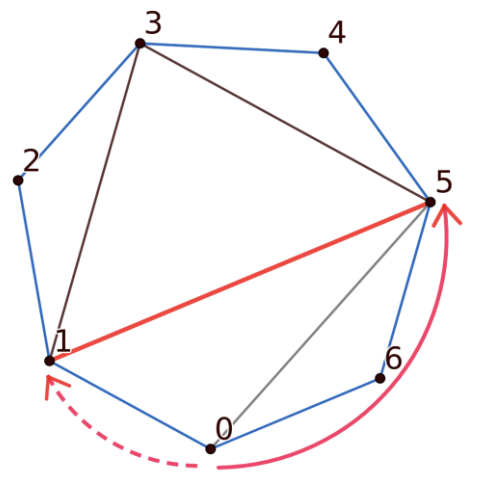시간 제한 메모리 제한 제출 정답 맞은 사람 정답 비율
1 초 512 MB 0 0 0 0.000%

## 문제

Anna drew a regular polygon with n vertices numbered from 0 to n − 1 in clockwise order. Later she triangulated it by drawing n − 3 diagonals that do not intersect each other. Except possibly where they touch at their endpoints. Diagonal is defined as straight lines between two different vertices that aren’t sharing a side.First, let's define the distance from the vertex A to the diagonal D. Suppose we start at vertex A and keep moving to the next vertex in clockwise order until we reach one of the endpoints of D. The number of sides traversed will be called left_distance. Similarly, right_distance is the number of sides traversed if we start at A and move counter-clockwise until reaching D. The distance from A to D is the maximum of left_distance and right_distance

In the example image the distance from vertex 0 to diagonal (1,5) is 2 with left_distance being 1 and right_distance being 2. As for diagonal (0,5) the distance from vertex 0 is 5, with left_distance=5 and right_distance=2.

Anna wants to make a challenge for Jacob. Jacob does not know which diagonals are drawn at all. He only knows the value of n, but can ask Anna multiple times about some pairs of vertices and she will tell him whether a diagonal is present between those vertices. Jacob’s goal is to find the closest (with distance defined as above) drawn diagonal from the vertex 0. You are going to help him to achieve his goal by asking Anna a limited amount of questions.

• 5 ≤ N ≤ 100

## 구현

You should implement the following function in your submission:

int solve(int n)
• This function is called exactly once by the grader
• n: number of vertices in the polygon
• This function should return the diagonal between some vertices a and b as an integer with value a ⋅ n + b
• If there are multiple diagonals with minimal distance you can return any of them

The above function can make calls to the following function:

int query(int x, int y)
• x: the first vertex number
• y: the second vertex number
• 0 ≤ x, y ≤ n − 1
• returns 1 if there is a diagonal between x and y and 0 otherwise

## 예제

Here is a sample input for grader and corresponding function calls. This input is shown in an image above.

The only line in the input has one integer: n

Sample grader will print each query call to the stdout and you have to manually answer it with 1 or 0.

Sample Input to grader Sample Calls
Calls Returns Calls Returns
7
solve(7)
query(0, 3)
0
query(0, 5)
1
query(1, 5)
1
solve returns 1 · 7 + 5 = 12
Correct!

## 점수

Let’s denote q as the amount of question you used on a single test. Additionally, w = n·(n-3)/2.

• If you ask an invalid question or guess incorrectly you will receive 0% of points for a test
• If w < q you will receive 0% of points for a test
• If n < q ≤ w you will receive 10 + 60 · (w-q)/(w-n) of points for a test
• If q ≤ n you will receive 100% of points for a test

## 제출할 수 있는 언어

C++17, C++14, C++20, C++14 (Clang), C++17 (Clang), C++20 (Clang)

## 채점 및 기타 정보

• 2800점 이상을 획득해야 를 받는다.
• 예제는 채점하지 않는다.